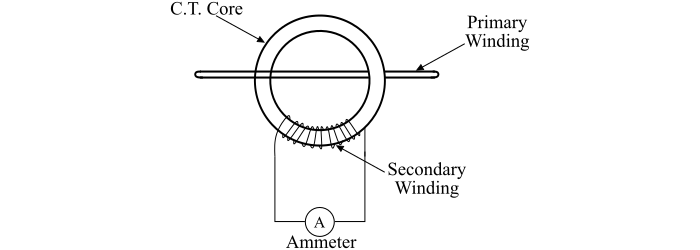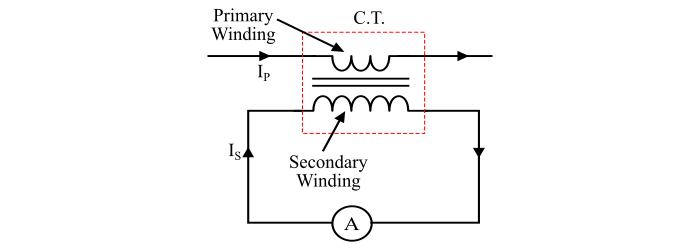# What is a Current Transformer?

A current transformer (C.T.) is an instrument transformer which is used for the protection and measurement purposes in a power system. The C.T. is primarily used to measure high alternating currents in a power system.

## Construction of Current Transformer

The magnetic core of the current transformers is made up of thin laminations of silicon steel. The primary winding of a CT has a single turn (also called bar primary) and carries full-load current whereas the secondary winding has large number of turns. Therefore, the current transformer is a voltage step-up and current step-down transformer (see the figure).The primary winding of the current transformer carries the current to be measured and the secondary winding carries a current proportional to the current to be measured. The measuring instrument (low range AC ammeter) is connected to the terminals of the secondary winding. Both the primary and secondary windings are insulated from the core and from each other.

If high degree of accuracy is desired in the measurement, then the core of the CT should be made up of high permeability nickel steel.

## Working of Current Transformer

The primary winding of the current transformer is connected in series with the line whose current is to be measured while the secondary winding connected to a low range (usually 0-5 A) AC ammeter (as shown in the figure).The relationship between the line current or primary current (IP) of the CT and the ammeter current or secondary current (IS) is given as follows,

$$\mathrm{𝑁_{𝑃}\:𝐼_{𝑃} = 𝑁_{𝑆}𝐼_{𝑆}}$$

$$\mathrm{\frac{𝐼_{𝑃}}{𝐼_{𝑆}}=\frac{𝑁_{𝑆}}{𝑁_{𝑃}}… (1)}$$

The equation (1) is known as the current transformation ratio or C.T. ratio i.e.

$$\mathrm{C. T.\:ratio =\frac{𝐼_{𝑃}}{𝐼_{𝑆}}… (2)}$$

Therefore, the line current is given by,

$$\mathrm{𝐼_{𝑃} = C. T.\:ratio × 𝐼_{𝑆} … (3)}$$

Hence, the line current is equal to the product of the CT ratio and the AC ammeter reading.

## Applications of Current Transformers

Some of the important applications of current transformers are as follows −

• The current transformers are used in measurement of high alternating currents in the power system.

• The current transformers are used in the power system protection applications such as to activate the protection relays.

## Why CT Secondary Should Never Be Kept Open?

The secondary winding of a current transformer should never be left open. When the current is flowing in the primary winding of a current transformer, its secondary winding should never be open circuited. When the current flows through the primary winding, a current also flows through the secondary winding and the MMF produced by each winding is equal and opposite.

Then, opening of the secondary winding under normal operation removes the opposing MMF produced by the secondary and allows the magnetic flux in the core of CT to reach a value that is determined only by the primary MMR. As a result of it, the flux in the core is greatly increased which leads the following harmful effects −

• The increased flux may saturate the magnetic core of CT which can leave the CT with a large value of residual flux and will seriously impair the accuracy in future.

• The flux in the core increases at a very high rate inducing a large voltage in the open circuited secondary winding of the current transformer. This high voltage may be sufficient to cause a danger to life and breakdown of the insulation.

Therefore, in view of the above discussion, if we have to remove to meter from the secondary circuit of a CT. We must first short-circuit the secondary and then remove the meter. The shortcircuit of the secondary winding may be removed after the secondary circuit is again closed.

## Numerical Example

A current transformer of 100/5 A is used to measure current in a transmission line. If the ammeter reads 4 A, then what is the current in the power line?

Solution

$$\mathrm{Line\:current,\:𝐼 = CT\:ratio × Ammeter \:reading}$$

$$\mathrm{\Rightarrow\:𝐼 =\frac{100}{5}× 4 = 80 \:A}$$